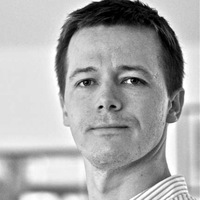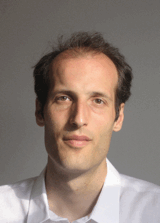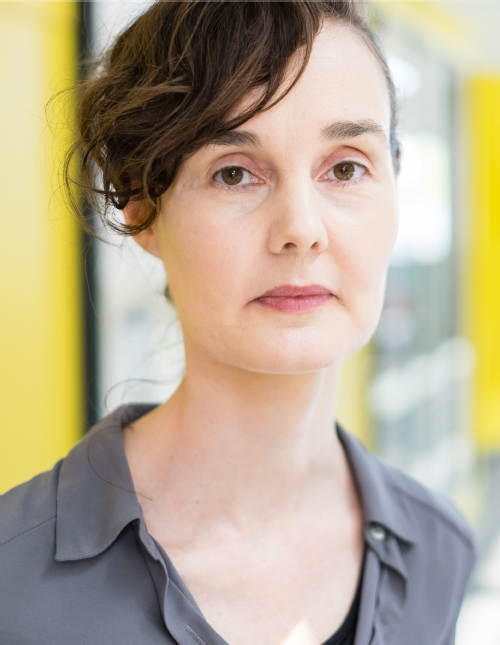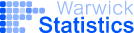# Probabilistic Numerics for Differential Equations - 21st April 2015

##Scope

Numerical algorithms, such as methods for the numerical solution of integrals and ordinary differential equations, as well as optimization algorithms can be interpreted as estimation rules. They estimate the value of a latent, intractable quantity – the value of an integral, the solution of a differential equation, the location of an extremum – given the result of tractable computations (“observations”, such as function values of the integrand, evaluations of the differential equation, function values of the gradient of an objective). So these methods perform inference, and are accessible to the formal frameworks of probability theory. They are learning machines.

Taking this observation seriously, a probabilistic numerical method is a numerical algorithm that takes in a probability distribution over its inputs, and returns a probability distribution over its output. Recent research shows that it is in fact possible to directly identify existing numerical methods, including some real classics, with specific probabilistic models.

Interpreting numerical methods as learning algorithms offers various benefits. It can offer insight into the algebraic assumptions inherent in existing methods. As a joint framework for methods developed in separate communities, it allows transfer of knowledge among these areas. But the probabilistic formulation also explicitly provides a richer output than simple convergence bounds. If the probability measure returned by a probabilistic method is well-calibrated, it can be used to monitor, propagate and control the quality of computations.

Further links and references for probabilistic numerics can be found at www.probabilistic-numerics.org.

SpeakersDr. Philipp Hennig, Emmy Noether Group Leader at the Max Planck Institute for Intelligent Systems, Tübingen. Philipp is a leading researcher in the emerging field of Probabilistic Numerics (PN). His research has established formal foundations for PN in areas that include optimisation, differential equations and experimental design.Prof. Martin Hairer, Regius Professor of Mathematics at the University of Warwick. Martin's research has contributed to our theoretical understanding of stochastic partial differential equations (SPDEs) and led to the development of new Taylor-like approximation schemes for SPDEs. He was recently awarded a prestigious Fields Medal in recognition of his achievements in this area.Dr. Catherine Powell, senior lecturer in Applied Maths and Numerical Analysis in the School of Mathematics at the University of Manchester. Catherine's research covers numerical analysis and is centered on approximation schemes and efficient computational tools for finding approximate solutions to partial differential equations (PDEs). Her research has let to applications that include fluid flow in porous media, image processing and electromagnetics.Dr. Søren Hauberg, Technical University of Denmark. Søren's background is in machine learning and computer vision research, where he works with geometric models of observed data. Søren's research tends to follow two directions: using geometric constructions to design models, or model constraints on data using geometry.Dr. Patrick Conrad, University of Warwick. Patrick recently joined Warwick from M.I.T. where he developed theoretical connections between the disciplines of Uncertainty Quantification and Monte Carlo Sampling. At Warwick, Patrick's work investigates the probabilistic solution of ordinary differential equation models.

Organisers

Correspondance should be sent to c.oates (at) warwick.ac.uk.